# How to Calculate and Solve for Heat Flux | Solidification of Metals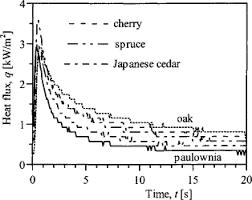The image above represents heat flux.

To compute for heat flux, three essential parameters are needed and these parameters are Thermal Conductivity (K), Density (ρ) and Heat Capacity at Constant Pressure (cp).

The formula for calculating heat flux:

q = K / ρcp

Where:

q = Heat Flux
K = Thermal Conductivity
ρ = Density
cp = Heat Capacity at Constant Pressure

Let’s solve an example;
Find the heat flux when the thermal conductivity is 21, the density is 12 and the heat capacity at constant pressure is 24.

This implies that;’

K = Thermal Conductivity = 21
ρ = Density = 12
cp = Heat Capacity at Constant Pressure = 24

q = K / ρcp
q = 21 / (12)(24)
q = 21 / 288
q = 0.0729

Therefore, the heat flux is 0.0729 W.

Calculating the Thermal Conductivity when the Heat Flux, the Density and the Heat Capacity at Constant Pressure is Given.

K = q (ρ cp)

Where:

K = Thermal Conductivity
q = Heat Flux
ρ = Density
cp = Heat Capacity at Constant Pressure

Let’s solve an example;
Find the thermal conductivity when the heat flux is 15, the density is 10 and the heat capacity at constant pressure is 14.

This implies that;

q = Heat Flux = 15
ρ = Density = 10
cp = Heat Capacity at Constant Pressure = 14

K = q (ρ cp)
K = 15 (10 x 14)
K = 15 (140)
K = 2100

Therefore, the thermal conductivity is 2100.

Calculating the Density when the Heat Flux, the Thermal Conductivity and the Heat Capacity at Constant Pressure is Given.

ρ = K / q x cp

Where:

ρ = Density
q = Heat Flux
K = Thermal Conductivity
cp = Heat Capacity at Constant Pressure

Let’s solve an example;
Find the density when the heat flux is 18, the thermal conductivity is 12 and the heat capacity at constant pressure is 4.

This implies that;

q = Heat Flux = 18
K = Thermal Conductivity = 12
cp = Heat Capacity at Constant Pressure = 4

ρ = K / q x cp
ρ = 12 / 18 x 4
ρ = 12 / 72
ρ = 0.167

Therefore, the density is 0.167.

Calculating the Heat Capacity at Constant Pressure when the Heat Flux, the Thermal Conductivity and the Density is Given.

cp = K / q x ρ

Where:

cp = Heat Capacity at Constant Pressure
q = Heat Flux
K = Thermal Conductivity
ρ = Density

Let’s solve an example;
Find the heat capacity at constant pressure when the heat flux is 10, the thermal conductivity is 20 and the density is 6.

This implies that;

q = Heat Flux = 10
K = Thermal Conductivity = 20
ρ = Density = 6

cp = K / q x ρ
cp = 20 / 10 x 6
cp = 20 / 60
cp = 0.33

Therefore, the heat capacity at constant pressure is 0.33.

Nickzom Calculator – The Calculator Encyclopedia is capable of calculating the heat flux.

To get the answer and workings of the heat flux using the Nickzom Calculator – The Calculator Encyclopedia. First, you need to obtain the app.

You can get this app via any of these means:

To get access to the professional version via web, you need to register and subscribe for NGN 2,000 per annum to have utter access to all functionalities.
You can also try the demo version via https://www.nickzom.org/calculator

Apple (Paid) – https://itunes.apple.com/us/app/nickzom-calculator/id1331162702?mt=8
Once, you have obtained the calculator encyclopedia app, proceed to the Calculator Map, then click on Materials and Metallurgical under Engineering.Now, Click on Solidification of Metal under Materials and Metallurgical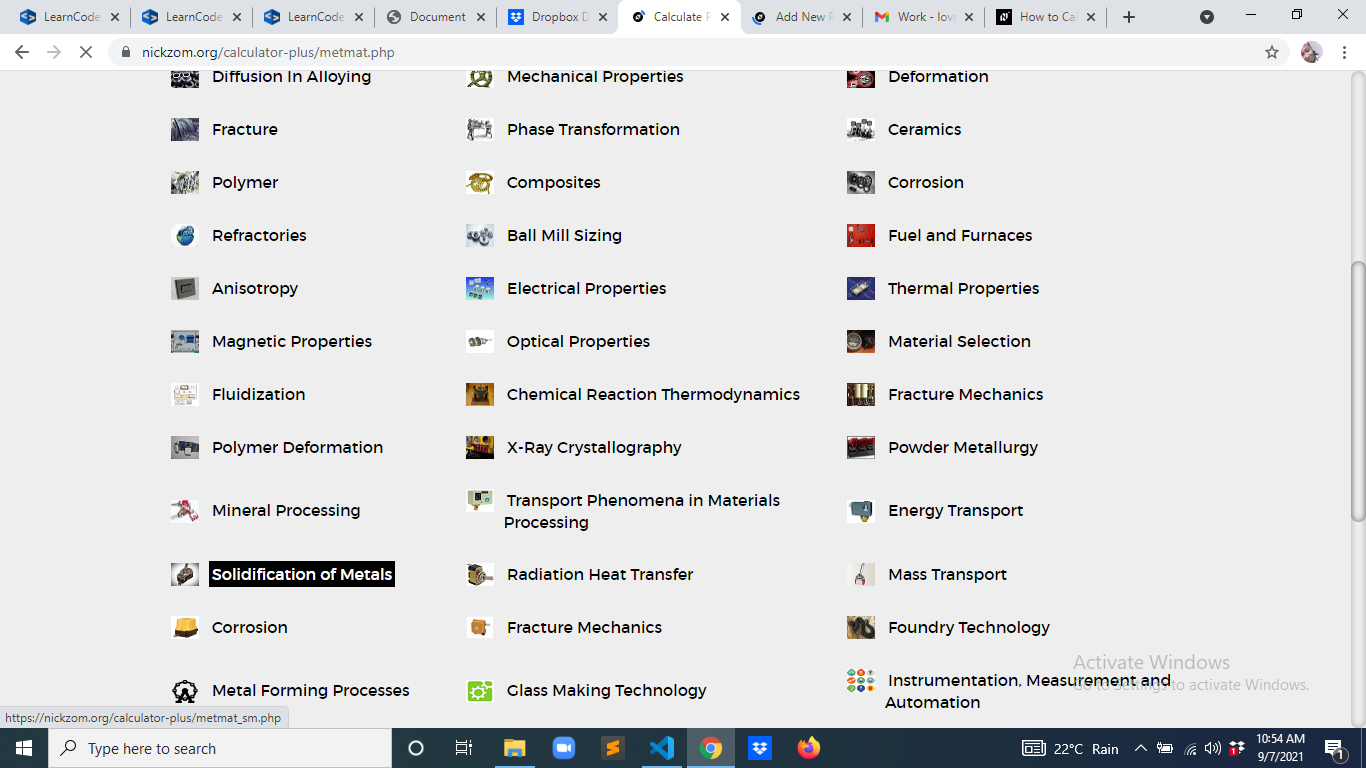Now, Click on Heat Flux under Solidification of Metal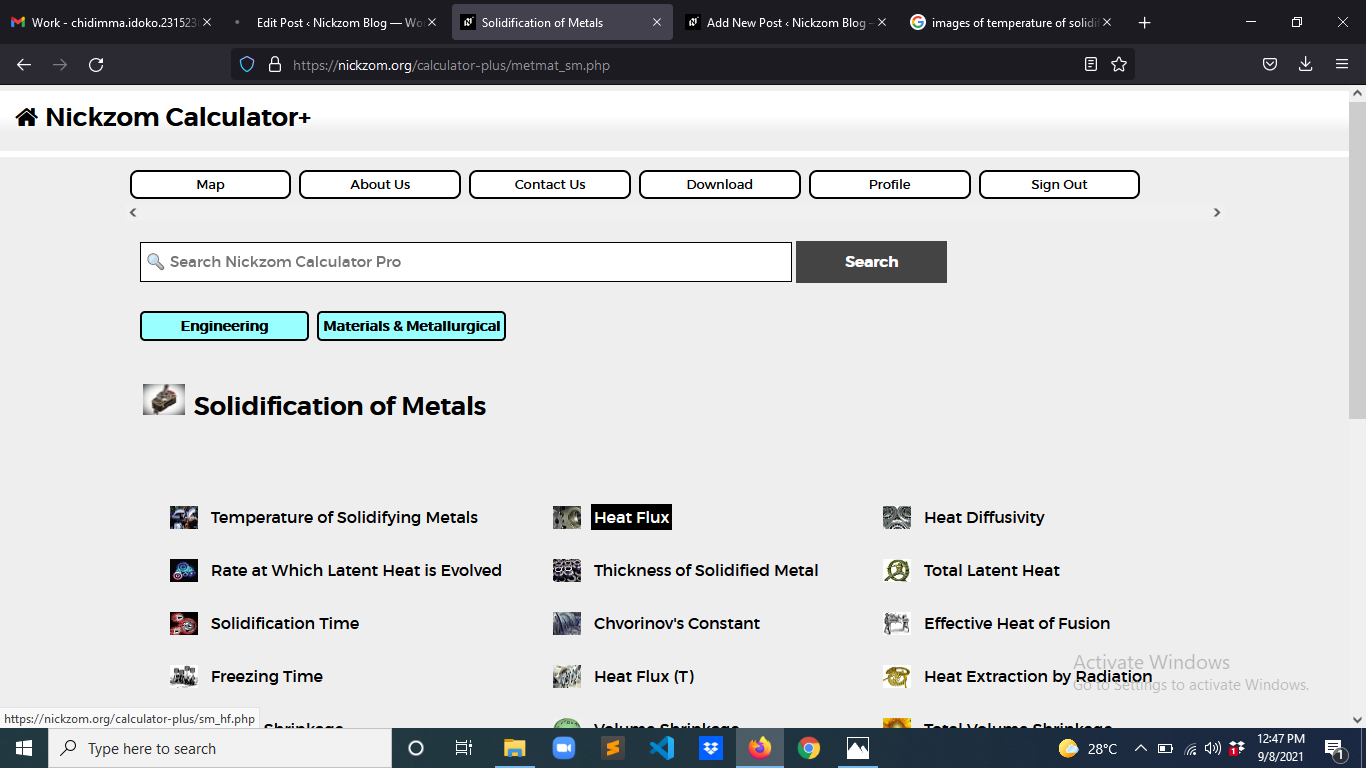The screenshot below displays the page or activity to enter your values, to get the answer for the heat flux according to the respective parameter which is the Thermal Conductivity (K), Density (ρ) and Heat Capacity at Constant Pressure (cp).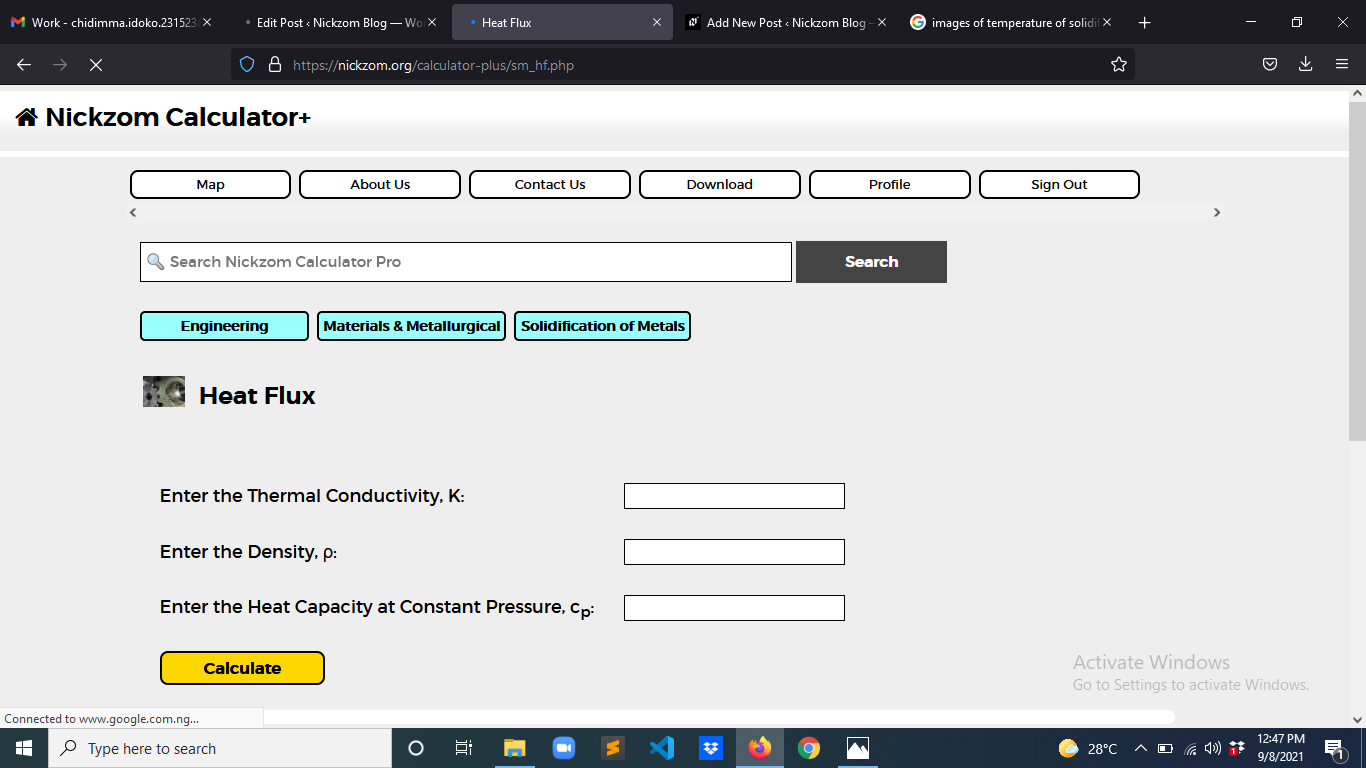Now, enter the values appropriately and accordingly for the parameters as required by the Thermal Conductivity (K) is 21, Density (ρ) is 12 and Heat Capacity at Constant Pressure (cp) is 24.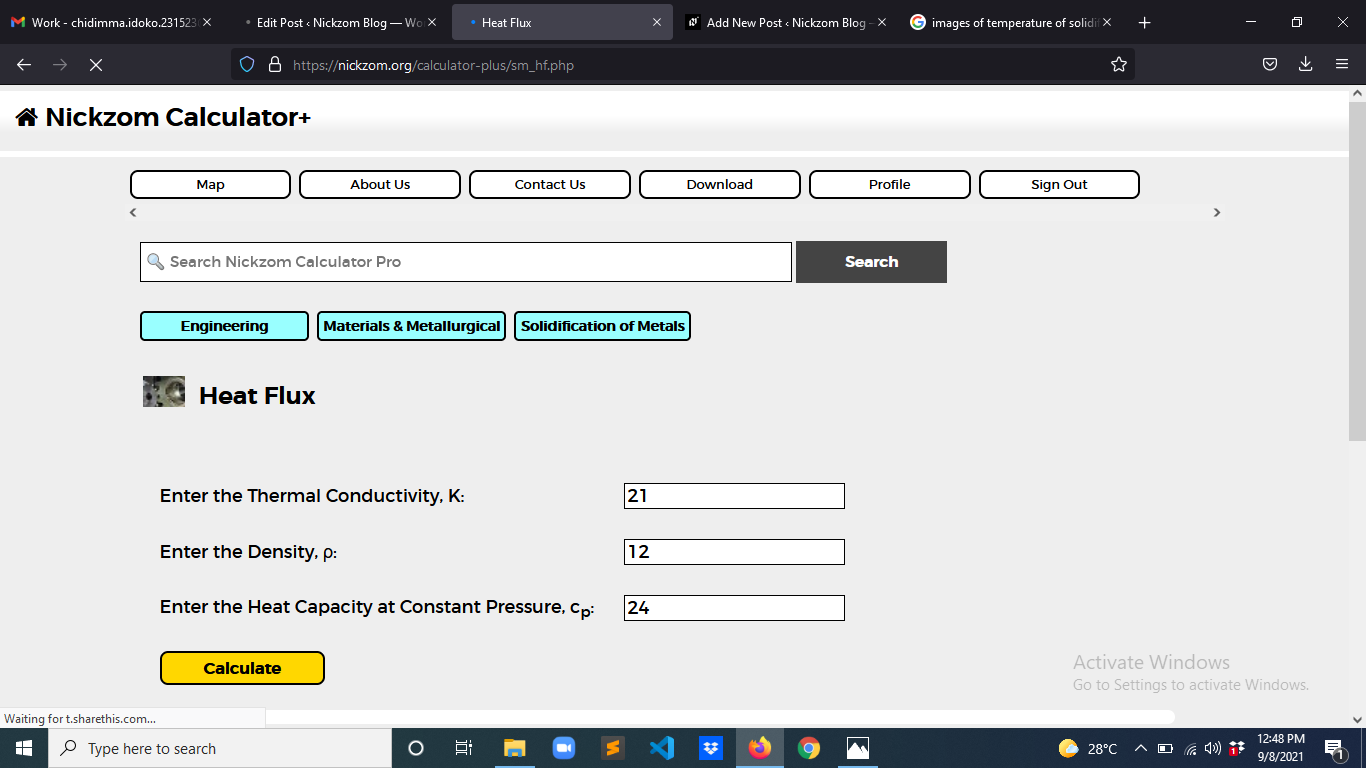Finally, Click on Calculate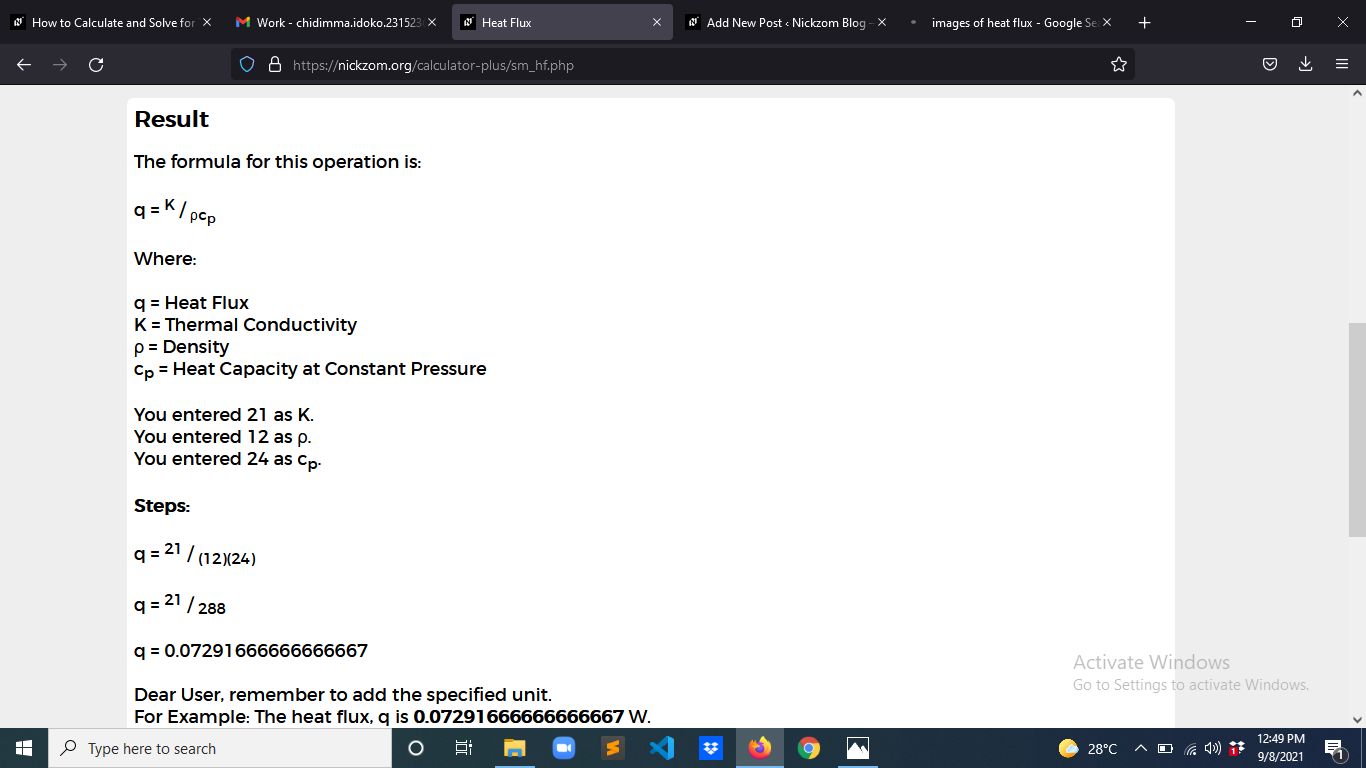As you can see from the screenshot above, Nickzom Calculator– The Calculator Encyclopedia solves for the heat flux and presents the formula, workings and steps too.